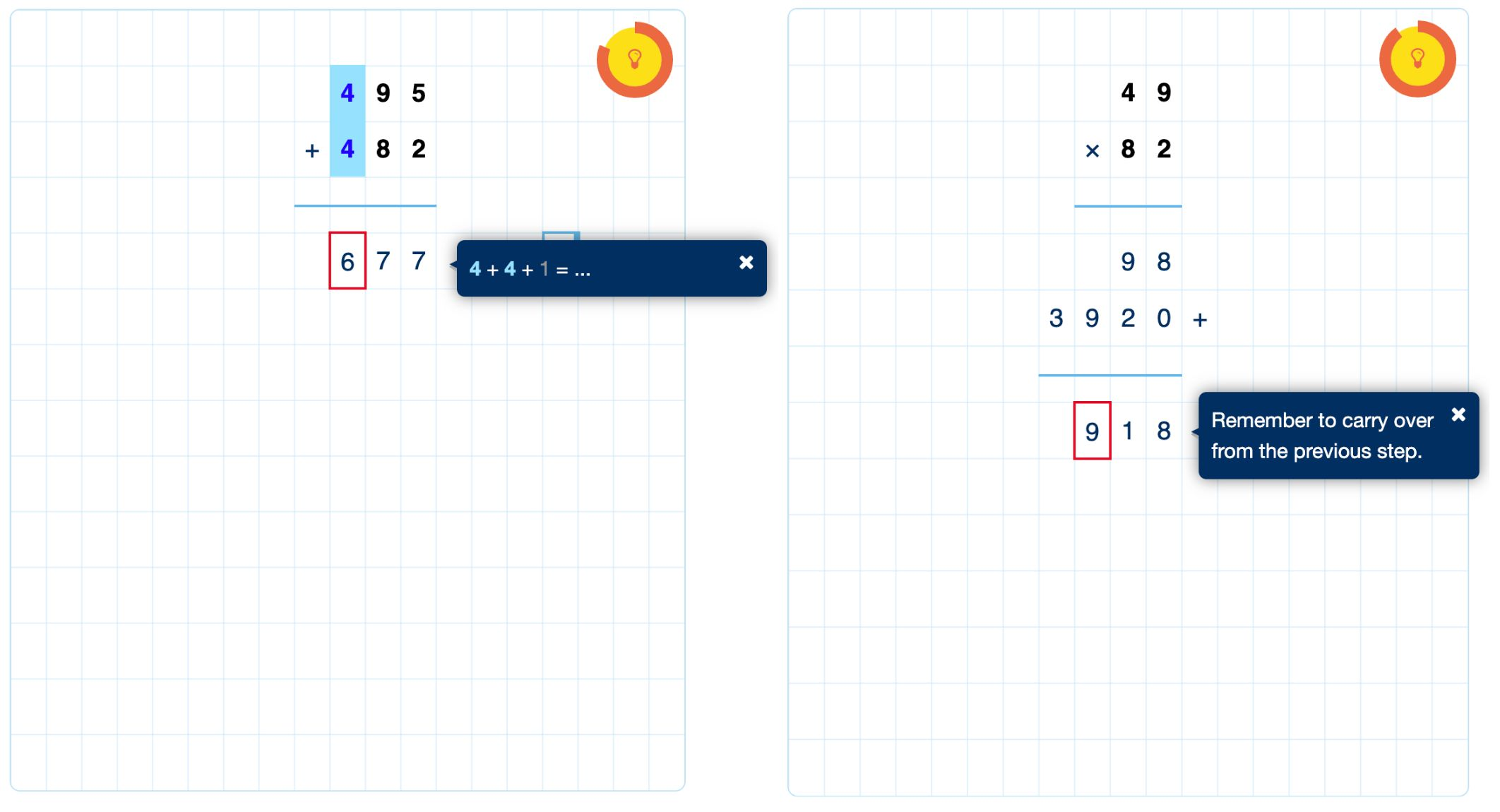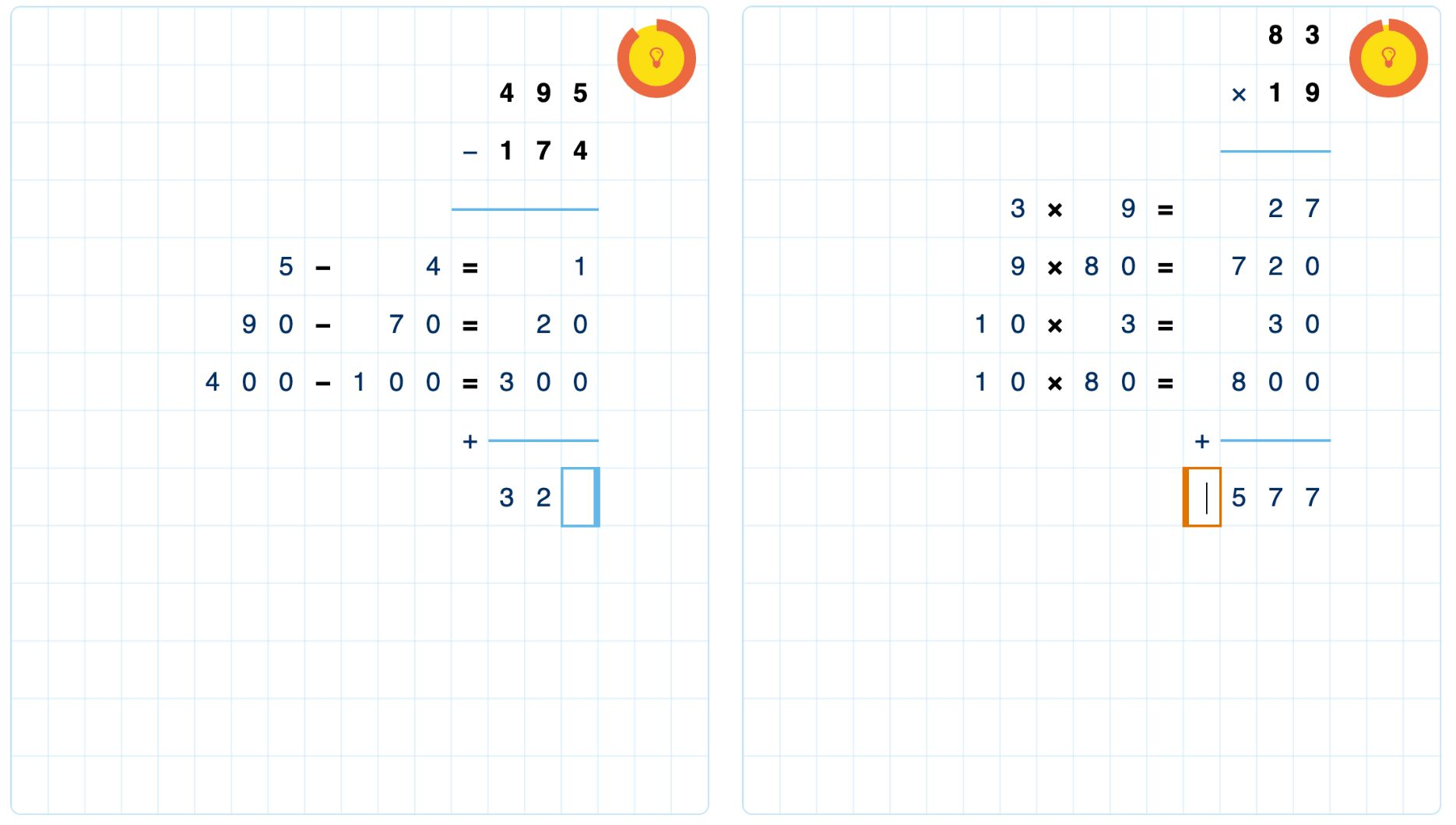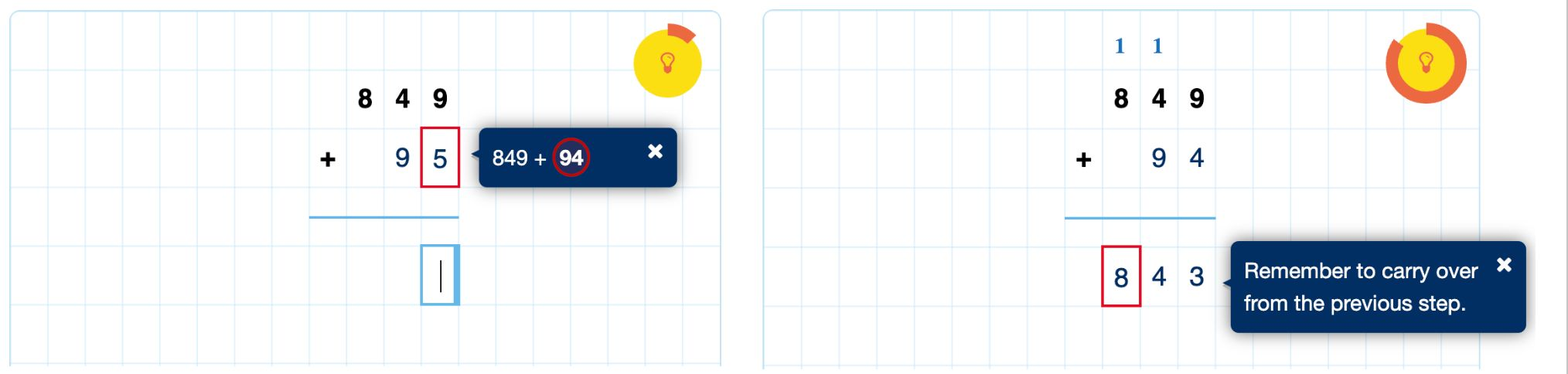# Written Arithmetic

## Multi-digit calculations with support

Written Arithmetic is a question type for multi-digit addition, subtraction, multiplication, and division. Students write their calculations similar to pencil and paper, while Algebrakit monitors their work in the background. A popup shows targeted feedback when students make a mistake.Figure: Multi-digit addition and multiplication with Written Arithmetic.

## Features

### Free input

Solving a math problem with Written Arithmetc feels like working with pen and paper. Instead of rigid input boxes, students can freely construct their solution on the canvas. The evaluation engine allows custom choices, such as

• Changing the order of the numbers for addition or multiplication
• Writing or skipping the carry or borrow numbers
• Using short or extended forms (see below).

The author can choose to set some of these choices in advance.

### Short and extended forms

Written Arithmetic supports various solution approaches. The short form is the most efficient method, using carry-over or borrowing. However, this is also the most difficult approach for students to learn. It is not uncommon that students first learn an extended form, where students calculate the result for each place value separately.Figure: Extended forms for subtraction and multiplication.

## Immediate evaluation and feedback

Algebrakit evaluates the students' work while they are working. Feedback is shown immediately and automatically whenever they make a mistake.

Note

It is possible to switch off automatic feedback by running the question in assessment mode. This is true for all of Algebrakit's question types.

Feedback uses as little language as possible to make Written Arithmetic suitable for young learners. The feedback displays as compact balloons that hover over the student's work.Figure: Feedback in Written Arithmetic.

## Live examples

### General Features

Like the other question types, Written Arithmetic can run in assessment mode to prevent students from seeing evaluation results, hints, or error feedback. This information is available during the review after the student submits the questions or tests.

Written Arithmetic supports random questions just like the other question types. Some exercises in the live example above are random.